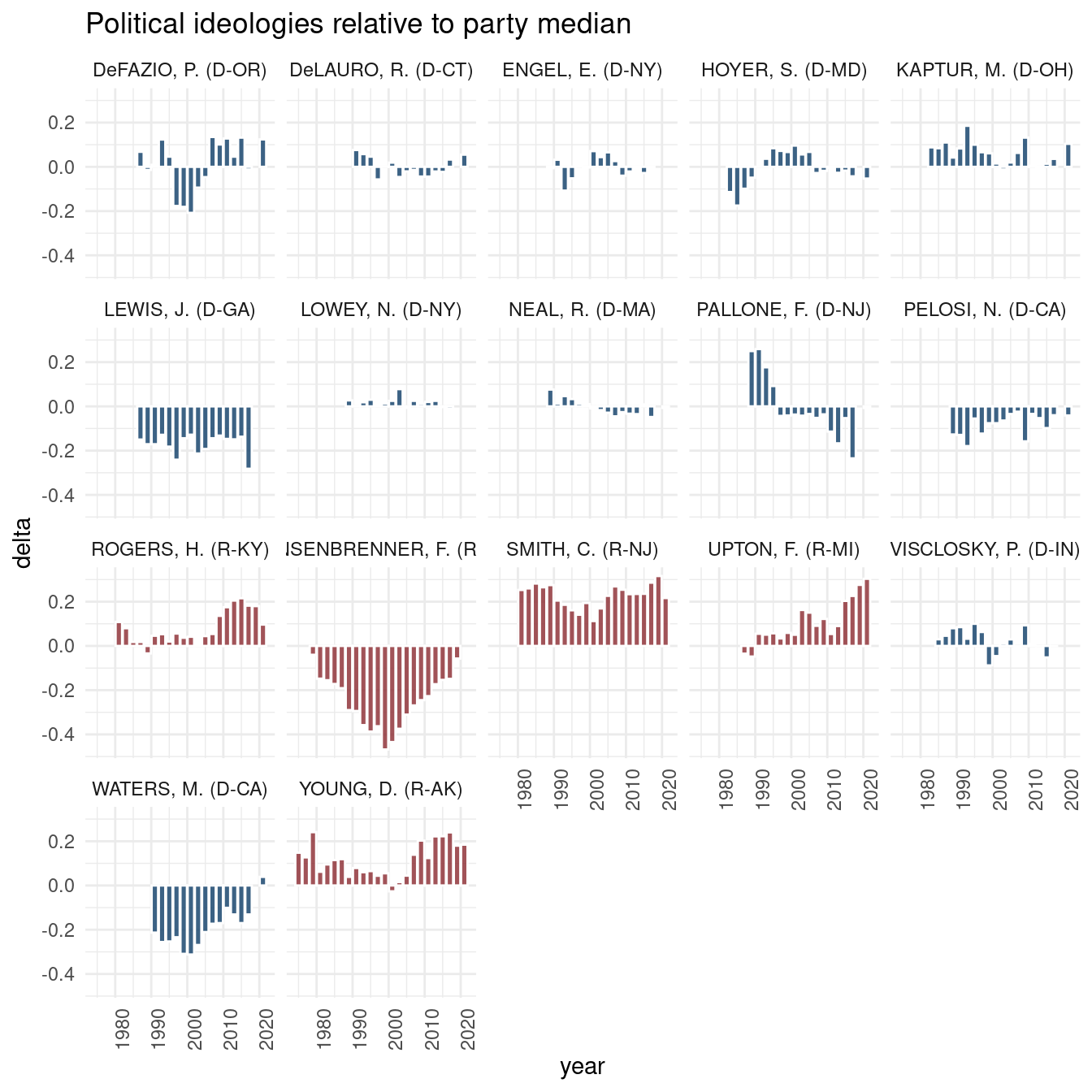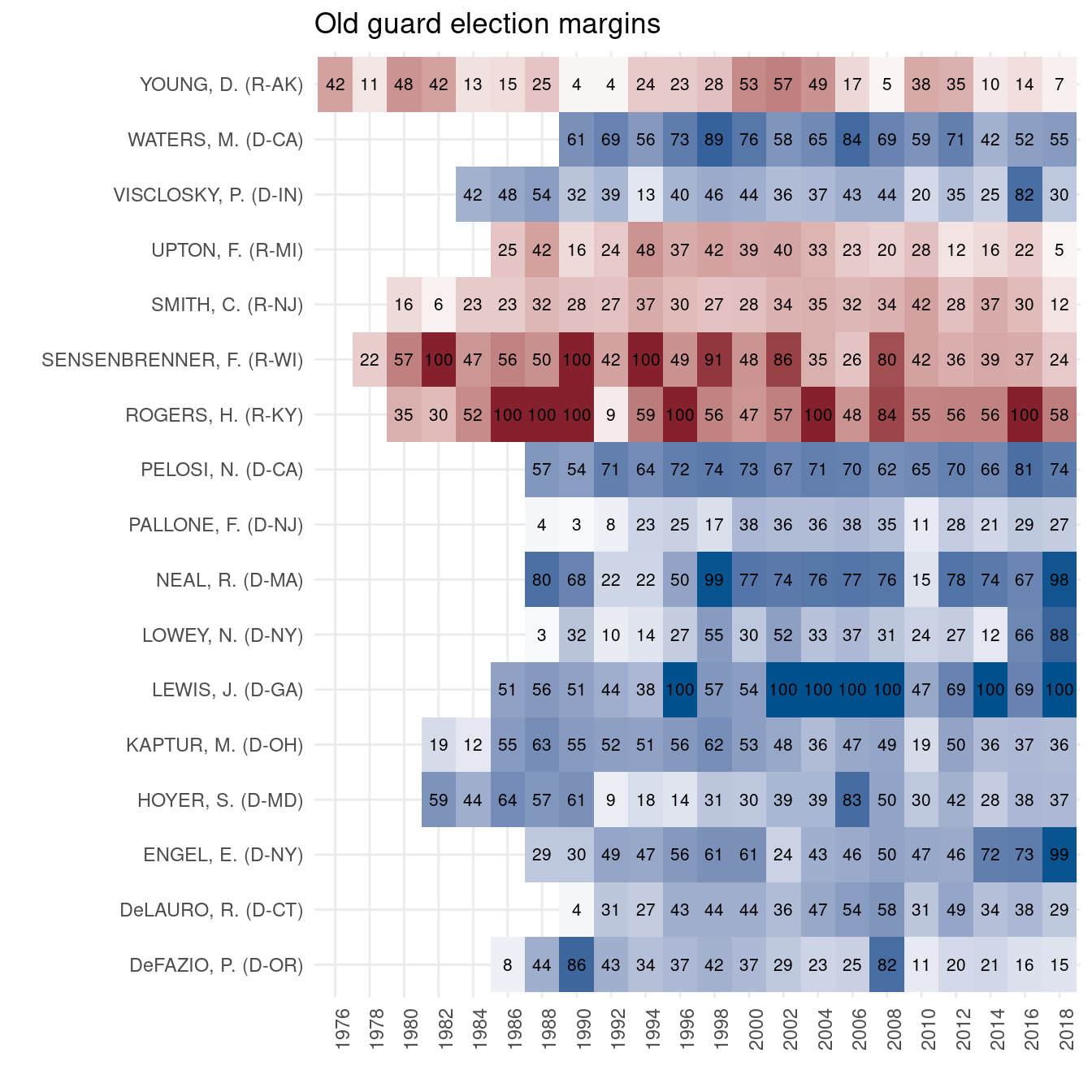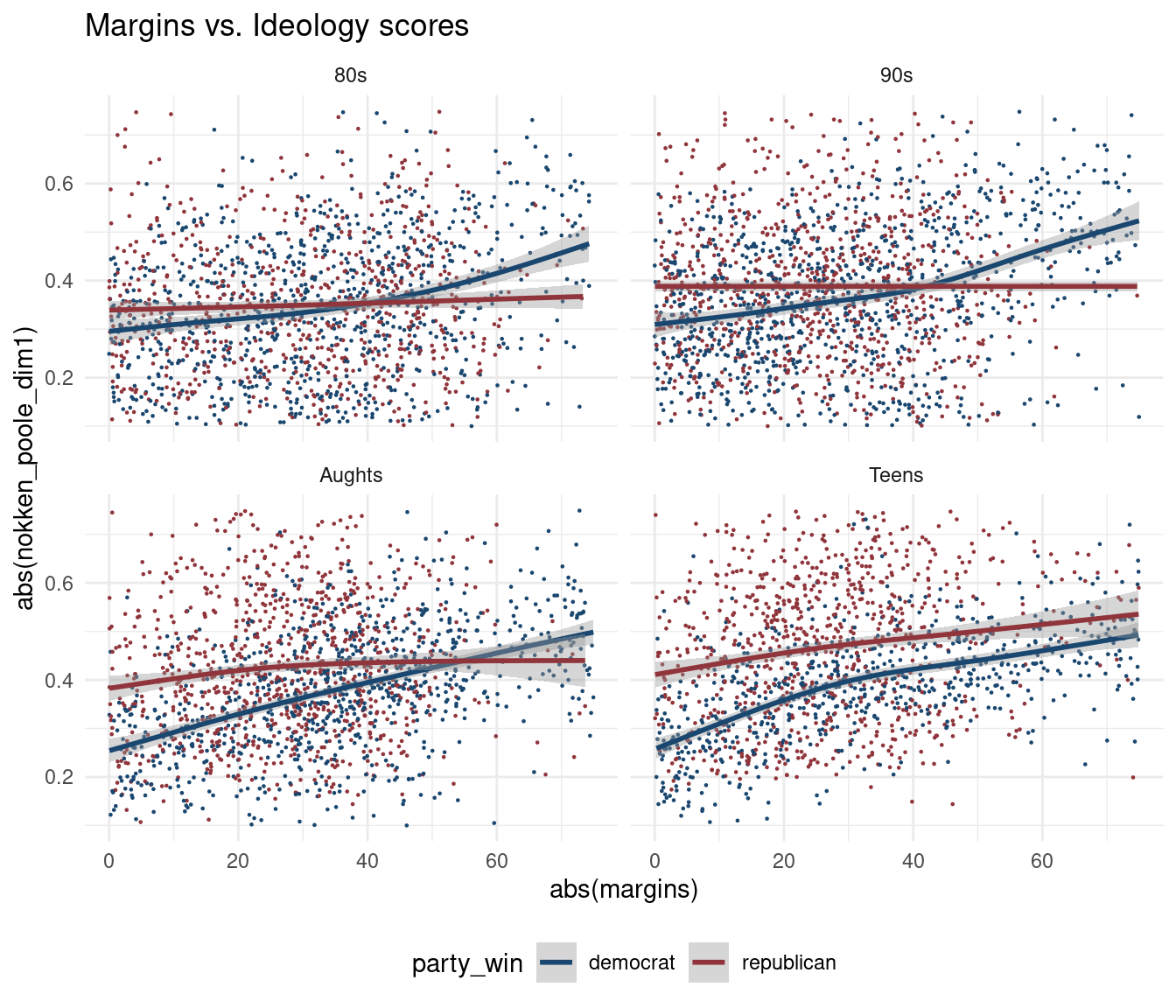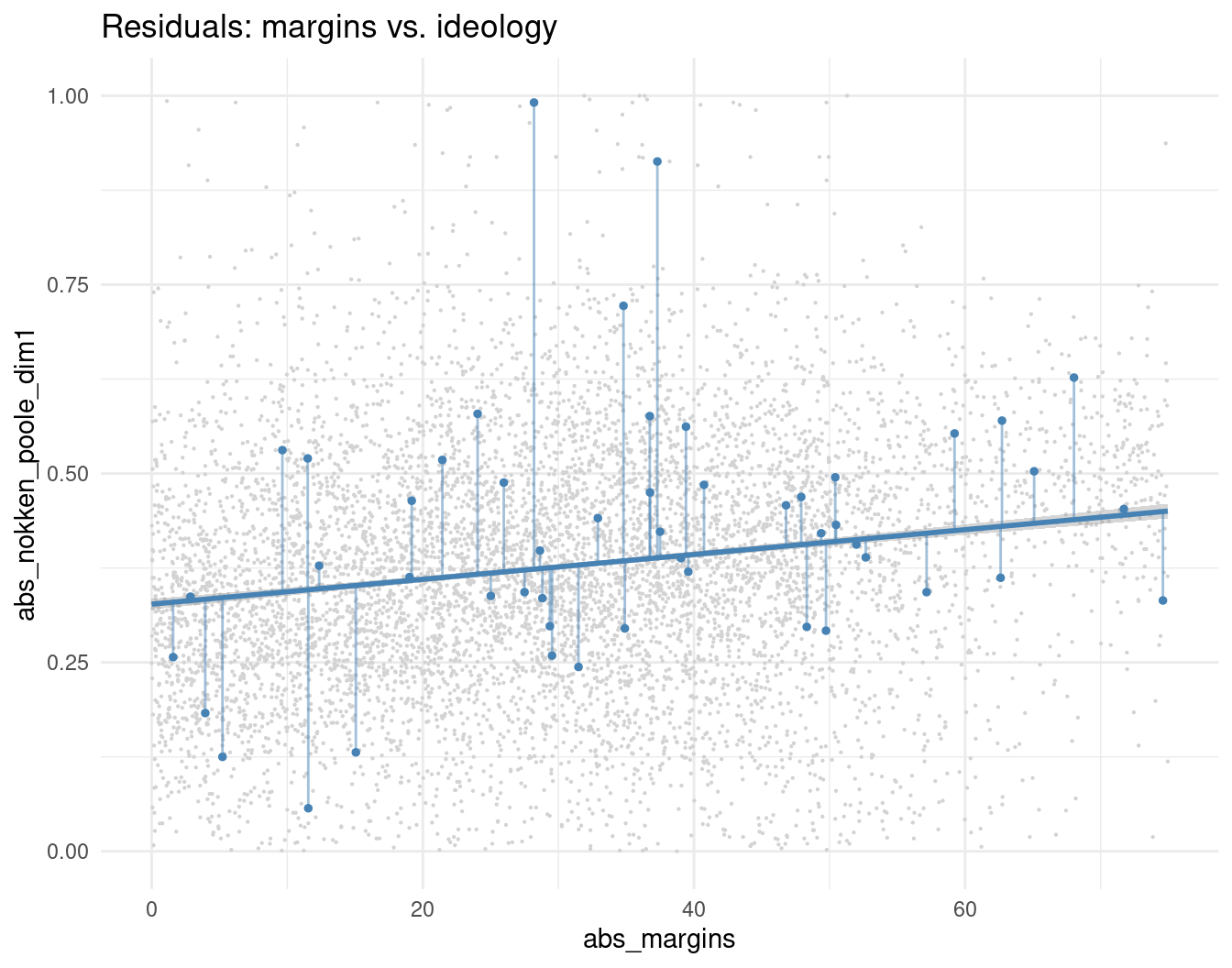# margins-of-victory, voting behavior & re-election

## Breifly

In this post, we take a couple of different historical perspectives on the US House of Representatives, investigating margins-of-victory, voting behavior, and re-election over the last 40 years. To introduce data sets, we first consider the body’s longest serving members, dubbed here the Old Guard.

Then we build a simple re-election model to investigate how the relationship between political ideology and margins-of-victory has influenced the likelihood of re-election in the House since 1976; among other things, model results demonstrate the relevance of voting in-step with one’s constituency.

## VoteView & DW-NOMINATE

To identify the longest serving members of the US House, and to explore their respective voting behaviors over time, we consult the VoteView project via the R package RVoteview. The download_metadata function provides easy access to a host of congressional member details, including Nokken-Poole ideology scores. These scores are congress-specific NOMINATE scores; in contrast, standard DW-NOMINATE scores are aggregated over the entire career of a lawmaker.

library(tidyverse)
chamber = 'house',
congress = 'all') 
##  "/tmp/RtmplTu5fW/Hall_members.csv"
x116 <- house_members %>%
filter(congress == 116, chamber == 'House') %>%
select(icpsr, chamber)

Next, we clean things up some, and derive/add several features to the data set, including the start year of a given congress and house member tenure (ie, the number of terms served) at the start of a given congress.

house_members <- house_members %>%
filter(last_means == 1 | is.na(last_means)) %>% # weirdnesses --
arrange(congress, bioname) %>%
group_by(bioname) %>%
mutate(congs_served = row_number()) %>%
ungroup() %>%

mutate(year = 2019 - (116-congress)*2,
party_code = ifelse(party_code == 200, 'R', 'D'),
age = year - born) %>%

group_by(congress, state_abbrev) %>%
mutate(n = length(district_code)) %>%
ungroup() %>%
mutate(district_code = ifelse(n == 1, 0, district_code)) %>%
select(-n)

## Longest-serving members of the US House

We filter the full data set to only members presently serving in the 116th House.

vnp <- house_members %>%
inner_join(x116) %>%
group_by(chamber, congress, party_code) %>%
mutate(med = median(nominate_dim1)) %>%
ungroup() %>%
mutate(label = gsub('([A-Za-z]*)(, )([A-Z])(.*$)', '\\1, \\3\\.', bioname), label = paste0(label, ' (', party_code, '-', state_abbrev, ')')) The longest serving members of the US House (currently holding office) since 1990. Focusing on members that have served consecutive, ie, uninterrupted, terms. longs <- vnp %>% group_by(icpsr, bioname, state_abbrev, party_code, label) %>% summarize(min = min(congress), max = max(congress), n = n()) %>% ungroup() %>% mutate(consec = ifelse(max - min + 1 == n, 'y', 'n'), since = 2019 - (n - 1) * 2, icpsr = as.character(icpsr)) %>% arrange(since) %>% filter(consec == 'y' & since < 1990) %>% select(icpsr, bioname, state_abbrev, party_code, label, since)  The Old Guard is presented in the table below. These members have held office since 1975 ~ 1989. longs %>% select(-label) %>% #filter(since < 1987) %>% DT::datatable(rownames = FALSE, options = list(dom = 't', pageLength = nrow(longs), scrollX = TRUE)) ## Longest-serving members & political ideology Here we consider the extent to which political ideology scores (may or may not) have shifted historically among the longest serving members of the US House. Again, we consider the Nokken-Poole first dimension scores made available via the Rvoteview::download_metadata function. The plot below summarizes these scores by congress for each of the old-guard House reps. Note that scores are presented relative to the party median for a given congress. For members of both parties, then, positive values reflect ideology scores that diverge from median towards 0, ie, scores are more moderate relative to median score for a given congress. for_plot <- vnp %>% inner_join(longs %>% select(bioname, since), by = c('bioname')) %>% mutate(delta = med - nokken_poole_dim1, delta = ifelse(party_code == 'D', -delta, delta)) %>% arrange(bioname) ggplot(data = for_plot, aes(x = year, y = delta, fill = factor(party_code))) + geom_bar(alpha = 0.85, color = 'white', stat = 'identity') + facet_wrap(~label) + theme_minimal() + theme(axis.text.x = element_text(angle = 90, hjust = 1)) + theme(legend.position = "none") + ggthemes::scale_fill_stata() + labs(title="Political ideologies relative to party median")So, each historical voting record a unique story. A more curious example above is the case of Frank Pallone Jr. Early on his career, he voted to the right of the Democratic party median; his voting behavior in more recent congresses has moved to the left of the party median. Also – and in general – some interesting post-9/11 patterns. ## Longest-serving members & election margins The next piece, then, is election margins. Returns for all congressional house races since 1976 are made available by the MIT Election Data and Science Lab; I have cleaned these data and included them in my uspols data package. house_returns <- uspols::uspols_medsl_house  The tile plot below details historical margins for members of the old guard. Margins for Republicans are calculated as the difference between % Republican vote and % Democratic vote; vice versa for Democrats. A nifty plot, and one that may be more interesting for districts with a bit more swing. lrs <- house_returns %>% filter(icpsr %in% longs$icpsr)

for_margins_plot <- lrs  %>%
mutate(per = ifelse(party_win == 'republican',
republican, -democrat),
per = round(per),
marg = round(republican-democrat)) %>%
inner_join(longs, by = 'icpsr') %>%
select(label, year, congress, marg)

ggplot(data = for_margins_plot,
aes(x = factor(year),
y = label)) +
geom_tile(aes(fill = marg)) +
geom_text(aes(fill = marg,
label = abs(marg)),
size = 2.75) +
mid = "white",
high = scales::muted("#ae4952"),
midpoint = 0) +
theme_minimal() +
theme(legend.position = "none",
axis.text.x = element_text(angle = 90, hjust = 1)) +
xlab('') + ylab('') +
ggtitle('Old guard election margins')## A re-election model – logistic regression

Generally, we expect a reasonably strong relationship between political ideology scores & election margins. Slim margins means an ideologically divided/diverse constituency, which translates to more moderate/middle-ground voting behavior in congress – in theory. An ideologically less diverse constituency often translates into more extreme voting behavior.

So, has the relationship between margins-of-victory and voting behavior influenced the likelihood of re-election for House members historically?

Using election returns and ideology scores for the last twenty congresses, here we present a simple logistic regression model to get at this particular question. For good measure, we also consider party affiliation, house member tenure, and the decade in which the election took place.

### /Re-election as dependent variable

With independent variables largely in tow, our goal here is to re-structure the VoteView data set such that each row represents a re-election “event.” For each seat in each congress since 1976.

widely <- house_members %>%
filter(congress > 94) %>%
select(congress, state_abbrev, district_code, bioguide_id) %>% #party_code,
group_by(congress, state_abbrev, district_code) %>%
slice(1) %>%
group_by(state_abbrev, district_code) %>%
spread(congress, bioguide_id)

A sample of the re-election portion of the data set is presented below. The first row summarizes the re-election event for Kentucky’s 4th congressional district, where ti is the 97th Congress and ti+1 the 98th Congress. Columns t1 and t2 denote bioguide ids for the representative of KY-04 in congresses 97 and 98, respectively. So, incumbent representative S000669 (Marion Snyder) won re-election to the 98th congress, as denoted by a value of 1 in the re_elect column.

Important methods note: no distinction is made here between losing an election and not seeking re-elction, for example.

x <- c(3:24)
y <- list()
for (i in 1:length(x)-1) { y[i] <- data.frame(df = c(x[i], x[i+1])) }

house_transitions <- lapply(1:length(y), function(j) {
a1 <- widely[, c(1:2, y[[j]])]
a1$trans <- paste(colnames(widely)[y[[j]]], collapse = '-') colnames(a1)[3:4] <- c('t1', 't2') a1 }) %>% bind_rows() %>% na.omit %>% mutate(congress = as.integer(gsub('-.*$', '', trans)),
re_elect = ifelse(t1 == t2, '1', '0')) %>%
ungroup()

Next, we create a simple category for election decade, spanning from the 70s to the Teens.

h2 <- house_transitions %>%
rename(bioguide_id = t1) %>%

inner_join(house_members %>% select(congress, state_abbrev, district_code,
bioguide_id, age, congs_served, nokken_poole_dim1)) %>%
left_join(house_returns %>% mutate(district_code = as.numeric(district_code))) %>%
mutate(margins = republican - democrat) %>%
mutate(decade = case_when (year < 1980 ~ '70s',
year < 1990 & year > 1979 ~ '80s',
year < 2000 & year > 1989 ~ '90s',
year < 2010 & year > 1999 ~ 'Aughts',
year > 2009 ~ 'Teens')) %>%
filter(party_win != 'other') %>%
na.omit # mostly at-large -- 

The faceted plot below, then, summarizes the relationship between election margins and political ideology scores across four decades of voting in the US House. Some variation in the details of this relationship historically, especially among Republican House members, but a fairly consistent one in the aggregate.

h3 <- h2 %>%
filter(abs(margins) < 75)

h3 %>%
ggplot(aes(y = abs(nokken_poole_dim1),
x = abs(margins), color = party_win
))+

geom_point(size = 0.15)+ #
geom_smooth(se = T) + #method="lm", se=T
ggthemes::scale_color_stata() +
theme_minimal() +
theme(legend.position = "bottom") +
ylim (0.1, 0.75) +
labs(title="Margins vs. Ideology scores")So, how does this relationship influence the likelihood of re-election? Can I vote like a progressive in congress if I only won my district by a couple of points? ie, will my constituency re-elect me if my voting behavior is not reflective of their ideology, at least in part?

From this perspective, the plot below highlights some residuals from a simple regression model of ideology scores against election returns (here, not controlled for by decade). Points above the fitted regression line represent House members whose ideology scores were higher (ie, more extreme) than expected based on their election margins; points below the fitted regression line represent members voting more moderately than expected based on election margins.

Here, we treat these residuals as an independent predictor. And for simplicity, we transform residuals to absolute values; in this way, all deviation from fit is treated equally.

dset <- h3
fit <- lm(abs(nokken_poole_dim1) ~ abs(margins), data = dset)
fit1 <- fit %>% broom::augment() %>% janitor::clean_names()

l1 <- ggplot(fit1, aes(x = abs_margins, y = abs_nokken_poole_dim1)) +
geom_point(size = .1, color = 'lightgrey') +
geom_smooth(method = "lm", se = T, color = "steelblue")

set.seed(119)
fit2 <- fit1 %>% sample_n(50)

l1 +
geom_segment(data = fit2, aes(xend = abs_margins, yend = fitted),
color = 'steelblue', alpha = 0.5) +
geom_point(data = fit2, aes(x = abs_margins, y = abs_nokken_poole_dim1),
size = 1, color = 'steelblue') +
theme_minimal() + ggtitle('Residuals: margins vs. ideology')### /Results

Using logistic regression via glm, we fit our model below. Again, margins, NOMINATE scores, and residuals are all transformed to absolute values. N = 7,844.

dset$residuals <- unname(fit$residuals)
dset$party <- as.factor(dset$party_win)
dset$decade <- as.factor(dset$decade)
dset$re_elect <- as.factor(dset$re_elect)

mod <- glm(re_elect ~
party +
congs_served +
abs(margins) +
abs(nokken_poole_dim1) +
abs(residuals),
data = dset)

Coefficients are summarized below; variables with independent effects on the likelihood of re-election to congress ti+1 are highlighted. R2 value ~ 5%. So, lots of variation on the table, and presumably any number of relevant predictors absent from the model.

td <- broom::tidy(mod) %>%
mutate_if(is.numeric, round, 3)

colors1 <- which(td$p.value < .05 & td$statistic > 0)
colors2 <- which(td$p.value < .05 & td$statistic < 0)

td %>%
knitr::kable(booktabs = T, format = "html") %>%
kableExtra::kable_styling() %>%
kableExtra::row_spec(colors1, background = "#e4eef4") %>%
kableExtra::row_spec(colors2, background = "#fcdbc7")
term estimate std.error statistic p.value
(Intercept) 1.219 0.126 9.706 0.000
partyrepublican -0.121 0.061 -2.005 0.045
congs_served -0.078 0.007 -11.299 0.000
abs(margins) 0.022 0.002 11.965 0.000
abs(nokken_poole_dim1) 0.667 0.187 3.566 0.000
abs(residuals) -0.914 0.288 -3.171 0.002

## Thoughts

So, independent effects for both margins and ideology scores on the likelihood of re-election. Big margins at election ti mean a higher likelihood of re-election to congress ti+1; as do higher ideology scores during congress ti. The former speaks to the comforts of sitting in a safe seat. The latter could speak to any number of things, including the fact that fringe party members tend to be more well-known & more popular on social media, etc. In contrast – Old Guard excepted – longer-tenure in the House detracts from the likelihood of re-election.

Perhaps of most interest are the independent effects of the ideology residuals on the likelihood of re-election. Per the plot above, the further a representative’s voting behavior strays from that predicted by election margins, the less likely that representative is to get re-elected to congress ti+!. So, some evidence that House reps are being held to account.

Of course, lots of caveats. The model is obviously super simple, with lots of variation left to be explained. But hopefully some useful methods.

Share